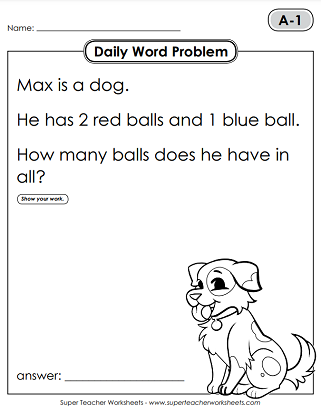# Daily Word Problems - Level A (1st Grade)

This page has sets of Daily Math Word Problems for 1st graders. Most problems require students to perform basic addition and subtraction, but there are also occasional problems related to place value and counting money.This file has 5 word problems, one for each day of the week. All problems require basic addition and subtraction and feature very simple vocabulary. If you prefer a full-color poster version of the problems, please click the "Alt" button below.
This file contains the next 5 word problems in the first grade set. All word problems have easy-to-read words, and require only basic addition and subtraction skills.
Solve five more simple addition and subtraction problems with simple, easy-to-read vocabulary.
Skills in this set include ordering numbers, operations, and very basic repeated addition.
Add and subtract to find the answers to math questions about hot dogs, triangles, toy cars, books, and toy tops.
These illustrated math stories require students to find simple sums and differences.
On these worksheets, students will answer more simple word problems. These include bats, jelly jars, camping, foxes, and calendars.
Count by twos starting with 2 (2, 12, 22, 32, 42...), practice repeated addition, as well as basic addition and subtraction.
Here are five more addition/subtraction word problems for 1st graders. Themes include horses, sandcastles, pie, pirates, and kittens.
Use addition and subtraction to find the answers to the next 5 daily word problems. One of the problems requires two steps.
Math Buzz: Daily Math Review

Our 'Math Buzz' series features daily review for all math skills.

More Daily Word Problems

On STW, you'll find a set of Daily Word Problems for all levels. Have you finished Level-A? Jump ahead to Level-B (2nd grade)!

## Sample Worksheet ImagesMy Account
Site Information